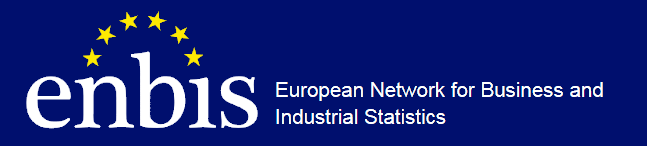#ENBIS-22 Trondheim Conference

26-30 June 2022
Europe/Berlin timezone

## Some optimization-theoretic issues in analysis of interval-valued data

27 Jun 2022, 11:30
30m
EL2

### EL2

Other/special session/invited session

### Speaker

Michal Černý (Prague University of Economics & Business)

### Description

Interval-valued data are often encountered in practice, namely when only upper and lower bounds on observations are available. As a simple example, consider a random sample $x_1, \dots, x_n$ from a distribution $\Phi$; the task is to estimate some of the characteristics of $\Phi$, such as moments or quantiles. Assume that the data $x_1, \dots, x_n$ are not observable; we have only bounds $\underline{x}_i \leq x_i \leq \overline{x}_i$ a.s. and all estimators and statistics are allowed to be functions of $\underline{x}_i, \overline{x}_i$ only, but not $x_i$. The analysis very much depends on whether we are able to make additional assumptions about the joint distribution of $(\underline{x}_i, x_i, \overline{x}_i)$ (for example, a strong distributional assumption could have the form
$\mathsf{E}[x_i|\underline{x}_i,\overline{x}_i]=\frac{1}{2}(\underline{x}_i+\overline{x}_i)$). Without such assumptions, a statistic $S(x_1, \dots, x_n)$ can only be replaced by the pair of tight bounds $\overline{S} = \sup\{S(\xi_1,\dots,\xi_n)|\underline{x}_i\leq\xi_i\leq\overline{x}_i\ \forall i\}$ and
$\underline{S} = \inf\{S(\xi_1,\dots,\xi_n)|\underline{x}_i\leq\xi_i\leq\overline{x}_i\ \forall i\}$. We report some of our recent results on the algorithms for the computation of $\underline{S}, \overline{S}$. In particular, when $S$ is the sample variance, it can be shown that the computation of $\overline{S}$ is an NP-hard problem. We study a method based on Ferson et al., which works in exponential time in the worst case, while it is almost linear on average (under certain regularity assumptions), showing that the NP-hardness result need not be too restrictive for practical data analysis.

Keywords interval data; statistical computing

### Primary authors

Michal Černý (Prague University of Economics & Business) Dr Sokol Ondřej (Prague University of Economics & Business)

### Presentation Materials

There are no materials yet.